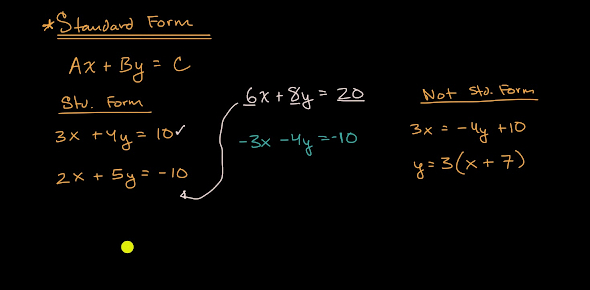# Standard Form - Form 4

6 Questions | Attempts: 1526
ShareSettingsWhile in school, mathematics is one of the subjects that is considered the toughest. However, enough practice makes it very simple. Below is a quiz on significant figures which will help in your practice and revision.

• 1.
The number of significant figures of 324 is
• A.

1

• B.

2

• C.

3

• D.

4

• 2.
The number of significant figures of 30024 is
• A.

3

• B.

4

• C.

5

• D.

6

• 3.
The number of significant figures of 30.24 is
• A.

2

• B.

3

• C.

4

• D.

5

• 4.
The number of significant figures of 0.00302 is
• A.

3

• B.

4

• C.

5

• D.

6

• 5.
The number 8000 has n significant figures. State the possible value of n.
• A.

0

• B.

1

• C.

5

• D.

6

• 6.
The number of significant figures of 0.040500 is
• A.

2

• B.

3

• C.

4

• D.

5

## Related TopicsBack to top
×

Wait!
Here's an interesting quiz for you.